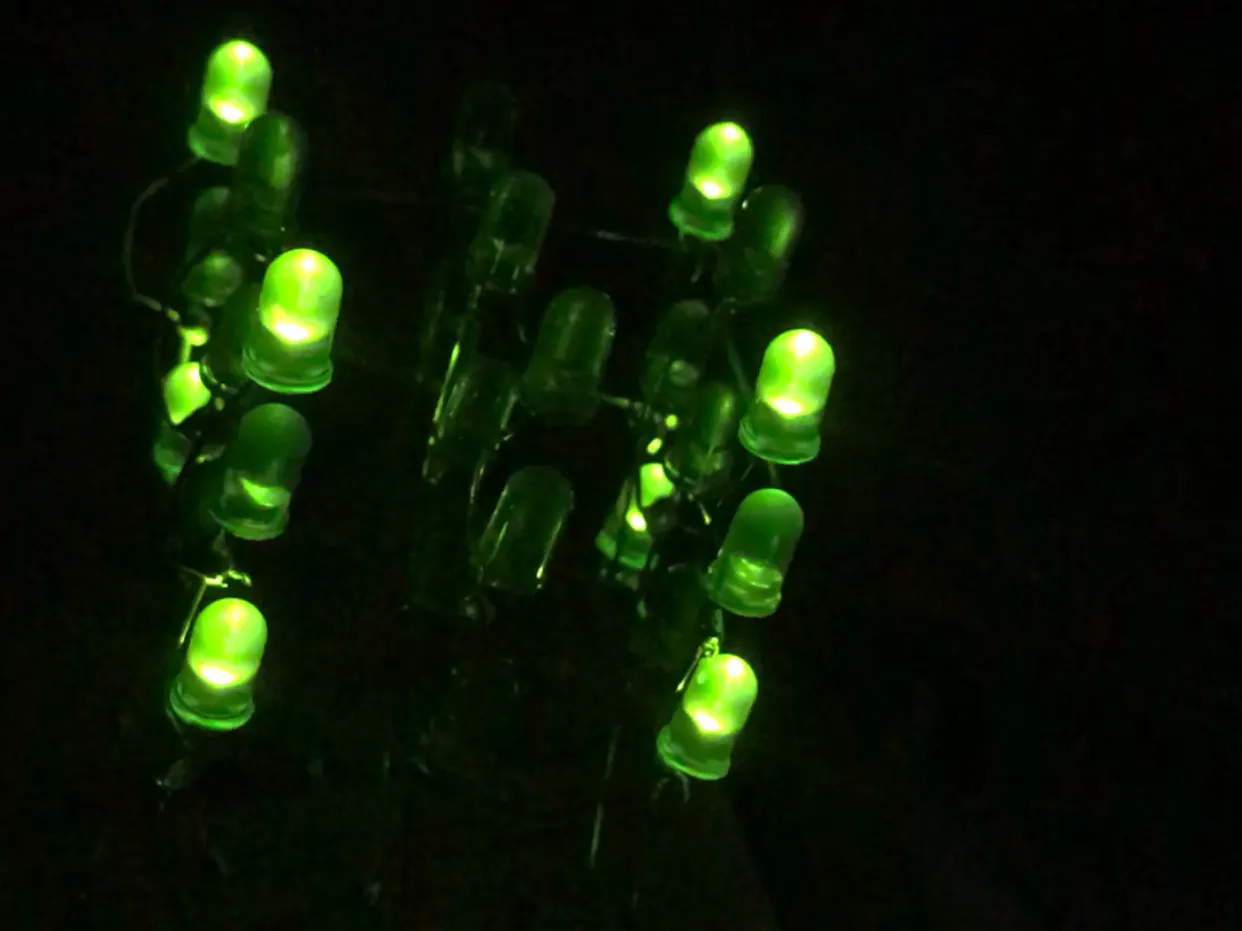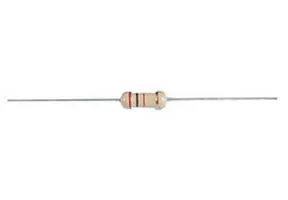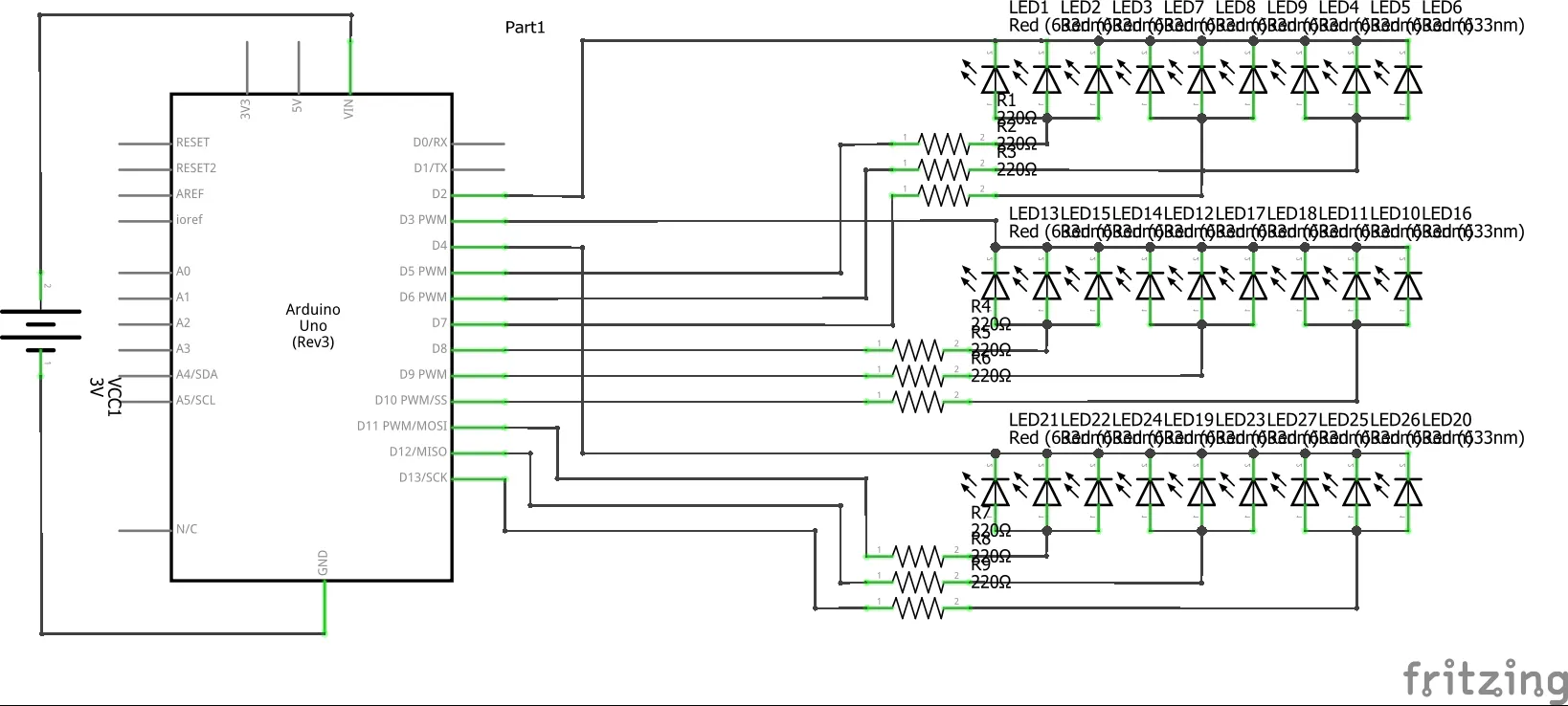# LED Cube

Arduino based 3D LED Cube (3x3x3) which can produce any light pattern with 27 LEDs.

BeginnerFull instructions provided2 hours32,406## Things used in this project

### Hardware componentsArduino UNO
×1LED (generic)
×27×1
 9V Battery
×1Jumper wires (generic)
×10Resistor 220 ohm
×9
 9V battery jack
×1

### Software apps and online servicesArduino IDE

### Hand tools and fabrication machinesSoldering iron (generic)

## Schematics

### LED Cube 3x3x3## Code

### LED_Cube.ino

Arduino
```void l(int x, int y, int z) {
if (y == 0) {
digitalWrite(2, LOW);
}
else if (y == 1) {
digitalWrite(3, LOW);

}
else if (y == 2) {
digitalWrite(4, LOW);
}
if (x == 0) {
if (z == 0) {
digitalWrite(9, HIGH);
}
else if (z == 1) {
digitalWrite(11, HIGH);
}
else if (z == 2) {
digitalWrite(13, HIGH);
}
}
if (x == 1) {
if (z == 0) {
digitalWrite(8, HIGH);
}
else if (z == 1) {
digitalWrite(10, HIGH);
}
else if (z == 2) {
digitalWrite(12, HIGH);

}
}
if (x == 2) {
if (z == 0) {
digitalWrite(6, HIGH);
}
else if (z == 1) {
digitalWrite(7, HIGH);
}
else if (z == 2) {
digitalWrite(5, HIGH);
}
}
}
void ol(int num) {
if (num == 1) {
digitalWrite(2, HIGH);
}
if (num == 2) {
digitalWrite(3, HIGH);
}
if (num == 3) {
digitalWrite(4, HIGH);
}
}
void onl(int num) {
if (num == 1) {
digitalWrite(2, LOW);
}
if (num == 2) {
digitalWrite(3, LOW);
}
if (num == 3) {
digitalWrite(4, LOW);
}
}
void off(int x) {
digitalWrite(5, LOW);
digitalWrite(6, LOW);
digitalWrite(7, LOW);
digitalWrite(8, LOW);
digitalWrite(9, LOW);
digitalWrite(10, LOW);
digitalWrite(11, LOW);
digitalWrite(12, LOW);
digitalWrite(13, LOW);
delay(x);
}
void setup() {
// put your setup code here, to run once:
pinMode(2, OUTPUT);
pinMode(3, OUTPUT);
pinMode(4, OUTPUT);
pinMode(5, OUTPUT);
pinMode(6, OUTPUT);
pinMode(7, OUTPUT);
pinMode(8, OUTPUT);
pinMode(9, OUTPUT);
pinMode(10, OUTPUT);
pinMode(11, OUTPUT);
pinMode(12, OUTPUT);
pinMode(13, OUTPUT);
}

void loop() {
// put your main code here, to run repeatedly:
onl(1);
onl(2);
onl(3);

l(1,0,1);
delay(500);
off(50);

l(0,0,0);
l(1,0,0);
l(2,0,0);
l(2,0,1);
l(2,0,2);
l(1,0,2);
l(0,0,2);
l(0,0,1);
delay(350);

ol(2);
ol(3);
delay(350);
off(0);

onl(1);
onl(2);
onl(3);

l(1,0,1);
delay(500);
off(50);

l(0,0,0);
l(1,0,0);
l(2,0,0);
l(2,0,1);
l(2,0,2);
l(1,0,2);
l(0,0,2);
l(0,0,1);
delay(350);

ol(2);
ol(3);
delay(350);
off(0);

onl(1);
onl(2);
onl(3);

l(1,0,1);
delay(500);
off(50);

l(0,0,0);
l(1,0,0);
l(2,0,0);
l(2,0,1);
l(2,0,2);
l(1,0,2);
l(0,0,2);
l(0,0,1);
delay(350);

ol(2);
ol(3);
delay(300);
off(0);

onl(1);
onl(2);
onl(3);

l(1,0,1);
delay(500);
off(50);

l(0,0,0);
l(1,0,0);
l(2,0,0);
l(2,0,1);
l(2,0,2);
l(1,0,2);
l(0,0,2);
l(0,0,1);
delay(350);

off(0);
ol(2);
l(0,0,0);
l(2,0,0);
l(2,0,2);
l(0,0,2);
delay(400);
off(100);
}
```